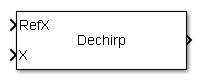# Dechirp Mixer

Dechirping operation on input signal

• Library:
• Phased Array System Toolbox / Detection

•## Description

The Dechirp block mixes the incoming signal from the `X` port with a reference signal incoming through the `RefX` port. The signals can be complex baseband signals. The input signal can be a matrix where each column is an independent channel. The reference signal is a vector. The reference signal is complex-conjugated and then multiplied with each signal column to compute the output.

## Ports

### Input

expand all

Reference signal, specified as a complex-valued M-by-1 vector.

Data Types: `single` | `double`
Complex Number Support: Yes

Input signal, specified as a complex-valued M-by-N matrix. Each column of `X` is an independent signal and is individually mixed with the signal input to the `RefX` port.

Data Types: `single` | `double`
Complex Number Support: Yes

### Output

expand all

Dechirped signal, returned as an M-by-N matrix. Each column of the `Port_1` port is the mixed output for the corresponding column of `X`.

Data Types: `single` | `double`
Complex Number Support: Yes

## Version History

Introduced in R2014b